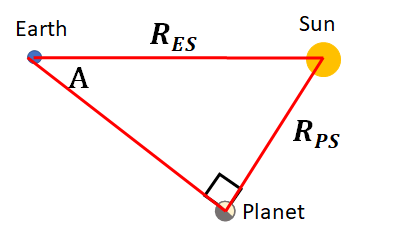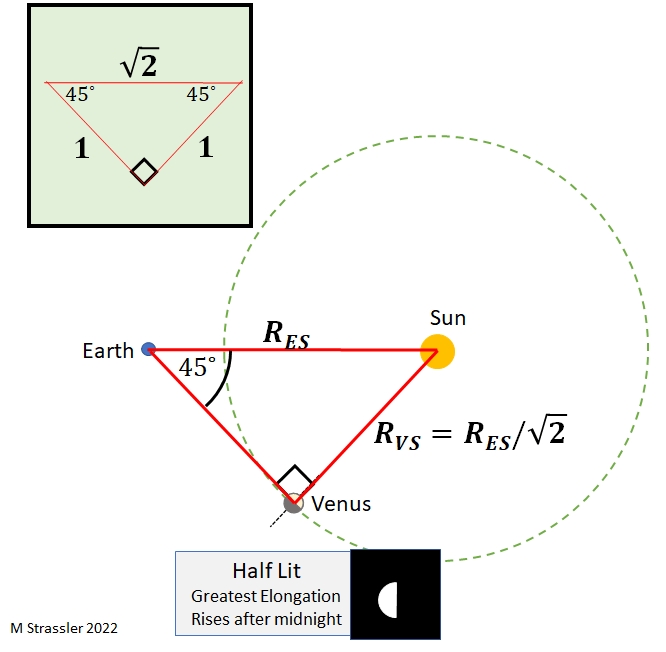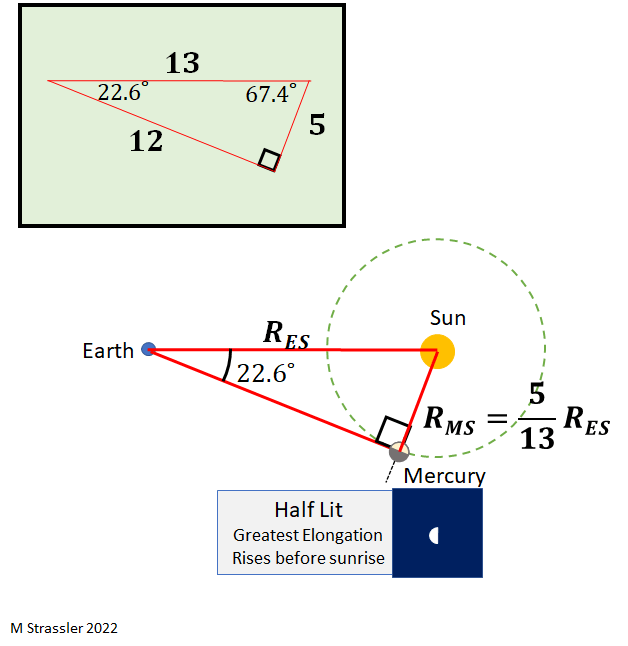# This Weekend, Measure the Distance to Venus Yourself

This Sunday, Venus reaches a special position from which it is easy to estimate roughly how large the average Venus-Sun distance (RVS) is relative to the average Earth-Sun distance (RES).  (I say “on average” because the Venus-Sun distance isn’t quite constant.  Venus’s orbit, like that of all the planets, isn’t quite circular.  But this is a small effect that we can ignore for the purpose of rough estimates.)

If you are a true diehard astronomy-geek, by all means get up at 5 or 5:30 in the morning on Sunday (or really any of the next few days) to check this directly.  I can assure you (since I have been up at that time recently, due to chronic insomnia more than astronomy-geekhood) that Venus looks absolutely gorgeous against the deep blue of the pre-dawn sky.  But if you have no intention on getting up that early, or clouds intervene, there’s a shortcut — on your phone.

### Greatest Elongation, Near-Circular Orbits, Half-Lighting and Right Angles

On Sunday, Venus moves to a position where, from Earth’s perspective, the angle between Venus and the Sun on the sky reaches its maximum.  This position is called “greatest elongation“, and it is reached twice per cycle, once in the evening sky and once in the morning.  If Venus’s orbit were perfectly circular, this would also be the moment when Venus appears half-lit; as we’ve been seeing in two recent posts (1,2), that’s an effect of simple geometry:

• if Venus’s orbit were circular, then at greatest elongation, the triangle formed by Earth, Venus and the Sun would be a right angle where Venus is located, and Venus would be half-lit.

This holds for Mercury too, as it would for any near Sun-orbiting planet.

Since Venus’s orbit isn’t quite circular, this isn’t precisely true; half lit and the right angle come together, but greatest elongation is off just a few days. This is a minor detail unless you’re an astronomy-geek, and won’t keep us from getting a good estimate of the Venus-Sun distance.

As illustrated in Figure 1, taken from this post which has more details about a planet’s overall cycle, any planet whose orbit is near-circular and lies closer to the Sun than Earth does can only be seen within a certain angle of the Sun. That assures that it can never be overhead at midnight, and so is only seen in the evening shortly after sunset or in the morning shortly before sunrise.Figure 1: As a near Sun-orbiting planet goes through its cycle, the angle between it and the Sun, as seen from Earth, never exceeds some maximum angle; it is always seen within the green wedge. It reaches that maximum at “greatest elongation”, when (if its orbit is near circular) it’s also approximately half-lit.

Figure 2 shows how the radius of a planet’s orbit affects the angle between the planet and the Sun at greatest elongation. A planet whose orbital radius is small is always within a small angle of the Sun; the right triangle at greatest elongation is narrow. A planet whose orbital radius is a larger fraction of the Earth-Sun distance can be seen at much larger angles, and the greatest elongation right triangle is much wider. Note also that the angle between the planet and the Sun, from Earth’s perspective, doesn’t change very quickly near greatest elongation. That means one can measure the angle within a few days of greatest elongation and still get pretty much the same answer.Figure 2: The larger a near Sun-orbiting planet’s orbit, the wider the angle between the planet and Sun, as seen from Earth, at greatest elongation. Specifically, the leftmost angle in the blue triangle between Earth, Sun and the planet is narrower than that in the green triangle; the red triangle is the widest. (Note this angle changes slowly near greatest elongation, and so can be measured within a few days of the actual maximum.)

### Finding the Radius of Venus’ OrbitFigure 3: at greatest elongation, the angle A reaches its maximum; the distance RPS from the planet to the Sun is RES sin(A) .

How do we apply this to get a distance from a planet to the Sun? The right triangle at greatest elongation has one of its sides stretching from the planet to the Sun, while its hypotenuse stretches from Earth to the Sun; see Figure 3 at right. The angle nearest the Earth on the triangle — let’s call it A — is the one that, from Earth’s perspective, is the angle between the planet and the Sun on the sky. As long as we can measure the angle A on the day of greatest elongation, we can use right-angle trigonometry to estimate the Planet-Sun distance: RPS = RES sin(A). And that’s gives us the answer. Very simple, and pretty accurate.

But we don’t need to pull out a calculator to do this for Venus, because it turns out, as we’ll check at the end of this post, that the angle A is pretty close to 45 degrees… just a bit larger. If the angle A were exactly 45 degrees, then the triangle would be a famous and easy one. Since every triangle on a flat surface has angles that add up to 180 degrees, a right triangle with one 45 degree angle has two 45 degree angles (90+45+45=180), and so this is also an isosceles triangle, whose two shorter sides have the same length.

Pythagoras tells us that if a right triangle’s sides have length a, b and c, where c is the length of the hypotenuse, then c2= a2 + b2. So if the two short sides of a right triangle both have length 1, then c2= 12 + 12 = 2; that is, the hypotenuse has length √2. From Figure 4 you can see that RES is the hypotenuse of the triangle we want, so RVS = RES/√2 = 0.707 RES.Figure 4: at greatest elongation, the Earth-Sun-Venus triangle is approximately that of triangle with angles of 90, 45 and 45 degrees, which has hypotenuse √2 larger than its sides (inset.) Since the Earth-Sun distance is the hypotenuse of the Earth-Sun-Venus triangle, this tells us the Venus-Sun distance is approximately √2 smaller than the Earth-Sun distance.

Since the angle of greatest elongation is slightly larger than 45 degrees, RVS will be just a little bigger than this. And indeed, if you look it up, you will find astronomers claim the ratio is 0.72. Now you know how they know.

To check that the Venus-Sun angle is about 45 degrees isn’t difficult, and I’ll tell you how to do it in a moment. But first, how would this same method work for Mercury, the other near Sun-orbiter?

In a few weeks, you can try the same trick with Mercury, which has its own moments of greatest elongation These occur often, because Mercury’s cycle is short, only 88 days from when it appears from behind the Sun until when it disappears behind the Sun again. The next one is April 29th, when the planet is visible in the evening sky; after that comes June 16th in the morning sky, and then again August 27th in the evening.

Because the distance between Mercury and the Sun RMS is so small, the Mercury-Sun angle at greatest elongation is quite a bit smaller than 45 degrees. Evidence that Mercury’s orbit is not so circular is that the angle can be as small as 18 degrees and as large as 28 degrees, for an average of 23 degrees.
Such is the claim, anyway. You can check this the same way as for Venus, using the methods I’ll describe below.

Now when the day comes and you do this measurement, then you’ll want to calculate the average Mercury-Sun distance. You can just compute sin(A), but alternatively, here’s a cute trick. You probably remember the right triangle whose sides are in the relation 3, 4, 5 (because 32 + 42 = 52). Well, there’s another less famous one with sides 5, 12, 13 (52+122=132), and the smallest angle in the triangle turns out to be 22.6 degrees, very close to what we want. So… I’ve drawn that triangle. And that tells you that RMS is, on average, about 5/13 RES. In decimals, 5/13 = 0.384…. Astronomers meanwhile claim that RMS is approximately 0.39 RES. … and now you know how to confirm it.Figure 5: the same as in Figure 4, now for Mercury. In this case the average angle of greatest elongation is about 23 degrees, the smallest angle in a 5-12-13 right triangle, so the average Mercury-Sun distance is about 5/13 of the Earth-Sun distance. The fact that this angle varies quite a bit from cycle to cycle, much more than the Venus-Sun angle, indicates the Mercury’s orbit is less circular and more elliptical than is Venus’s orbit.

### Measuring the Planet-Sun Angles

Up to now I’ve just told you what the Venus-Sun and Mercury-Sun angles are at greatest elongation. What’s the best way for you or me to actually measure them?

For Venus, it’s satisfying but somewhat challenging to measure it right on the sky, directly. That’s a little tricky, because it’s not easy (though it is possible) to see Venus and the Sun at the same time. Venus is easily seen before sunrise, but not just before or after. And it’s hard to guess exactly where the Sun is before it actually shows up. But if you keep track of where Venus is as sunrise approaches, you may be able to spot it with binoculars just after sunrise. Or you may be able to pinpoint it using an astronomy app on your phone, just as the Sun is rising, and find it with binoculars that way. Then you can directly measure the angle with a geometer’s compass, or you can settle for an intuitive estimate, using the fact that 45 degrees is exactly half a right angle. (Right angles are easy for humans to guesstimate, and it’s also easy for us to divide things in half.)

For Mercury, this method is really difficult. Mercury’s much less bright than Venus and more subject to the Sun’s glare, so it’s quite hard to measure the angle directly. Fortunately there’s another method, perhaps less satisfying but certainly much easier… and for cases where the planet’s up before sunrise, it’s less problematic if you like to sleep late. This involves using an astronomy app on your phone.

The app will tell you where any planet is relative to the Sun at any time, even right now. For instance, if you use the open source app Star Map, available for Android Phones, and point your phone at the Sun (though of course you can do this indoors, on a cloudy day, or even after sunset, since it’s calculating the Sun’s position rather than actually registering it), you’ll see something like the picture at right, which will show you the position of the Sun and all the planets that are nearby in the sky.Figure 6: A screen shot from the open source app Sky Map obtained today, two days before greatest elongation, showing the current location of planets and Sun as seen from Earth. As shown, a crude estimate of the Venus-Sun angle can be obtained by pretending the sky coordinates on the picture are flat rather than curved, and this leads (via Pythagoras) to an estimate of a Venus-Sun angle of about 48 degrees.

It happens that the planets form a somewhat unusual pattern right now. One part’s no accident: the planets and the Sun (and the Moon too, though it’s off screen) always lie nearly on one line, called the ecliptic. That feature arises from the fact that all the planet orbits and the Moon’s orbit roughly lie in an imaginary disk, as you can explore on this Nasa webpage; we can get back to the reasons for this another time. But the fact that so many of the planets are found between the Sun and Venus in the sky is a pure accident. It happens, but it’s not very common. It’s just a matter of where the planets happen to be distributed in three dimensional space, as projected onto the two dimensions of the sky from Earth’s perspective. Again, you can see how this looks in three dimensions on this Nasa webpage. This close arrangement will last only a few months, but it will make for a great fall of planet gazing, when several planets will reach the evening sky together.

Okay, let’s measure the angle between the Sun and Venus. The grid on the screen shot in Figure 6 is a little weird looking because it involves coordinates projected onto the sphere of the sky, whose details I don’t want to get into right now. Since the coordinates are curved, a right angle triangle doesn’t quite follow Pythagoras’ rules, but the triangle’s small enough that the approximation of using Pythagoras isn’t that bad, as long as we measure the two sides well. The grid is 10 degrees wide horizontally and 15 degrees wide vertically, so you can see that in the figure the Sun and Venus are about 14 degrees apart horizontally and about 46 degrees vertically. Using Pythagoras again, 142+462=48.12, so the angle measured this way seems to be about 48 degrees. That’s pretty close to the true angle, and close enough to the 45 degrees that we used for a quick estimate, confirming that the Venus-Sun distance is about 70% of the Earth-Sun distance.

Maybe around April 29th we’ll try this for Mercury.